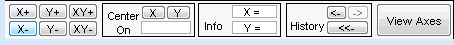## Continuous Wavelet Analysis of Noisy Sinusoid Using the Wavelet Analyzer App

This example shows how to use Continuous Wavelet 1-D tool to analyze a noisy sinusoidal signal.

1. Start the Continuous Wavelet 1-D Tool. From the MATLAB® prompt, type

```waveletAnalyzer ```

The Wavelet Analyzer appears.Click the Continuous Wavelet 1-D menu item.

The continuous wavelet analysis tool for 1-D signal data appears.At the MATLAB command prompt, type

`load noissin;`
In the Continuous Wavelet 1-D tool, select File > Import from Workspace. When the Import from Workspace dialog box appears, select the `noissin` variable. Click OK to import the noisy sinusoid signal.

The default value for the sampling period is equal to 1 (second).

3. Perform a Continuous Wavelet Transform.

To start our analysis, let's perform an analysis using the `db4` wavelet at scales 1 through 48, just as we did using command line functions in the previous section.

In the upper right portion of the Continuous Wavelet 1-D tool, select the `db4` wavelet and scales 1–48.4. Click the Analyze button.

After a pause for computation, the tool displays the coefficients plot, the coefficients line plot corresponding to the scale a = 24, and the local maxima plot, which displays the chaining across scales (from a = 48 down to a = 1) of the coefficients local maxima.5. View Wavelet Coefficients Line.

Select another scale a = 40 by clicking in the coefficients plot with the right mouse button. See step 9 to know, more precisely, how to select the desired scale.

Click the New Coefficients Line button. The tool updates the plot.6. View Maxima Line.

Click the Refresh Maxima Line button. The local maxima plot displays the chaining across scales of the coefficients local maxima from a = 40 down to a = 1.Hold down the right mouse button over the coefficients plot. The position of the mouse is given by the Info frame (located at the bottom of the screen) in terms of location (X) and scale (Sca).7. Switch from scale to Pseudo-Frequency Information.

Using the option button on the right part of the screen, select Frequencies instead of Scales. Again hold down the right mouse button over the coefficients plot, the position of the mouse is given in terms of location (X) and frequency (Frq) in Hertz.This facility allows you to interpret scale in terms of an associated pseudo-frequency, which depends on the wavelet and the sampling period..

8. Deselect the last two plots using the check boxes in the Selected Axes frame.9. Zoom in on detail.

Drag a rubber band box (by holding down the left mouse button) over the portion of the signal you want to magnify.10. Click the X+ button (located at the bottom of the screen) to zoom horizontally only.The Continuous Wavelet 1-D tool enlarges the displayed signal and coefficients plot (for more information on zooming, see Connection of Plots in the Wavelet Toolbox User's Guide).As with the command line analysis on the preceding pages, you can change the scales or the analyzing wavelet and repeat the analysis. To do this, just edit the necessary fields and click the Analyze button.

11. View normal or absolute coefficients.

The Continuous Wavelet 1-D tool allows you to plot either the absolute values of the wavelet coefficients, or the coefficients themselves.

More generally, the coefficients coloration can be done in several different ways. For more details on the Coloration Mode, see Controlling the Coloration Mode.

Choose either one of the absolute modes or normal modes from the Coloration Mode menu. In normal modes, the colors are scaled between the minimum and maximum of the coefficients. In absolute modes, the colors are scaled between zero and the maximum absolute value of the coefficients.

## SupportGet trial now